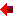IB / Pre-University / University MathObjective: The Diploma Programme for Grade 12 students. It is a globally recognized program that better prepares students for university. Not only does the IB Programme promote highly educated students, but it also encourages globally aware and involved individuals. Who Should Attend: This Math is intended for IB students. Also suited for whoever going to university must have higher level Knowledge in Math. Even good for Students who are studying Math (Calculus, Functions, Algebra & etc..) in 1st and 2nd year at university. Textbook: NallPro refer to a lot of University, IB and High School Textbooks. NallPro will give the Notes, Examples with explanation and Worksheets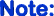Programme Modify according to students needs

 Math Tuition Fee & Period Fee Base on Monthly, Not by Class or Hourly! 1 Class Weekly! 2 Classes Weekly! IB SL \$150 Monthly \$230 Monthly IB HL / Pre-University Math \$160 Monthly \$250 Monthly According to my knowledge & previous experience weekly 1 class enough for most of the student (70% or More...) . Note: If a student got 60% or less better take 2 Classes per week. For Hourly Rates: \$50 (Minimum 2 hours charge per session)

 NallPro's Sample Worksheets! For IB & University Math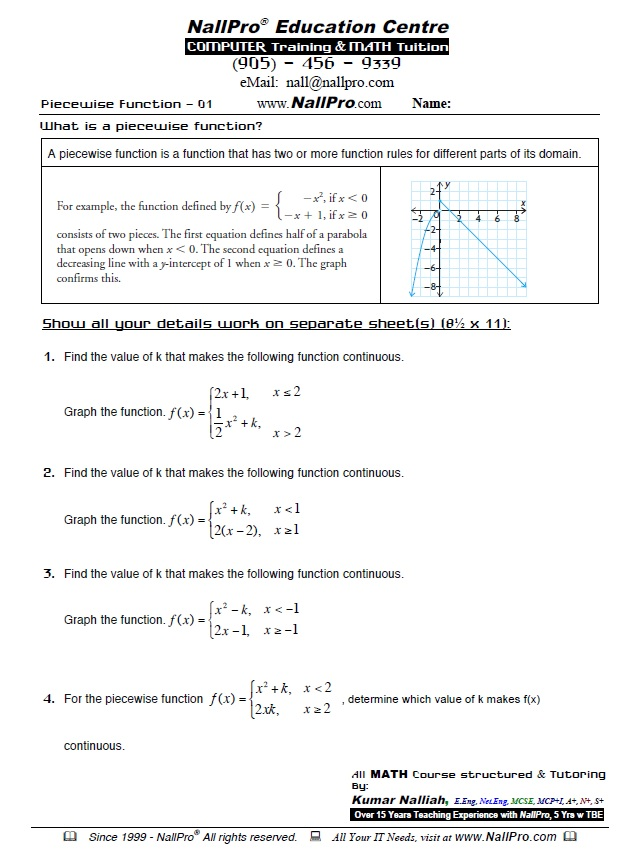Work Sheet 1PDF Printable version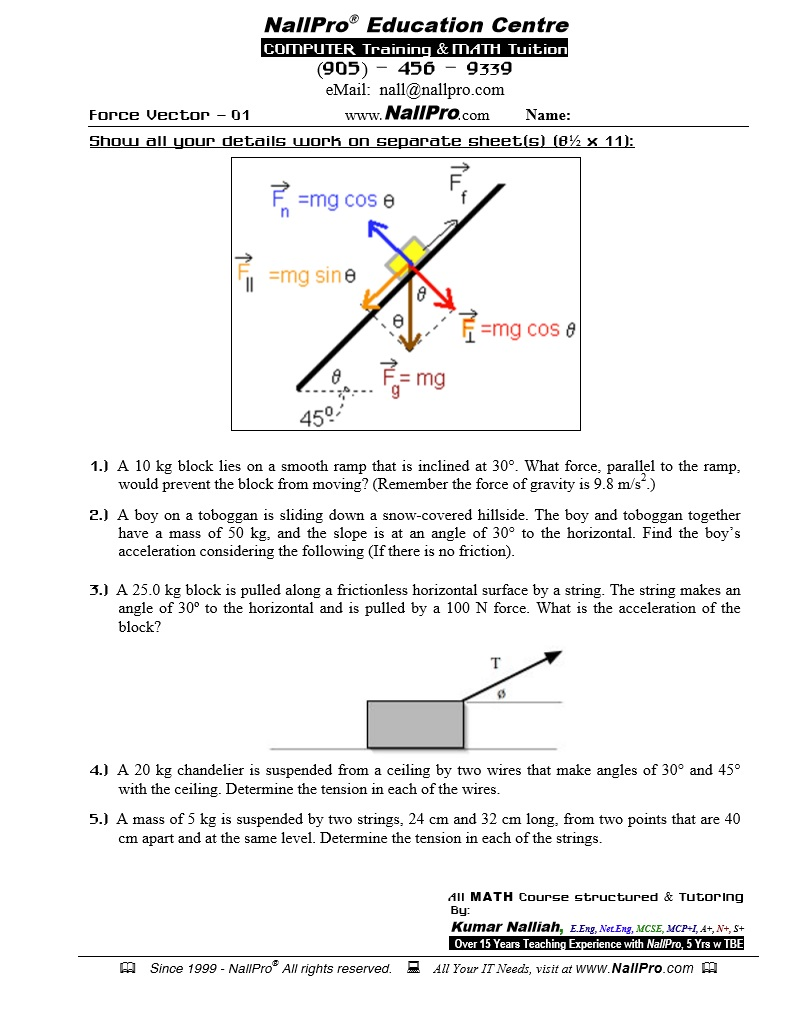Work Sheet 2PDF Printable version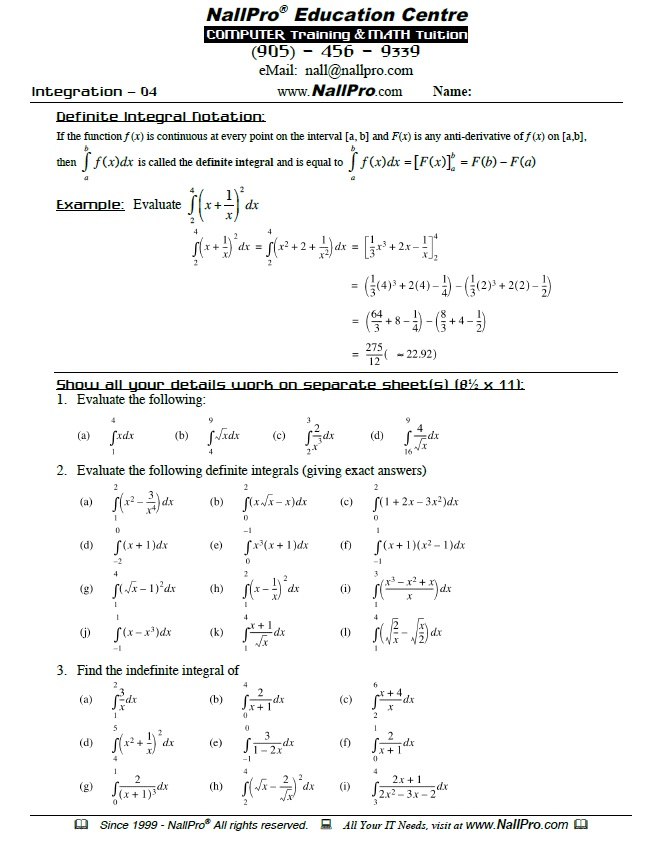Work Sheet 3PDF Printable version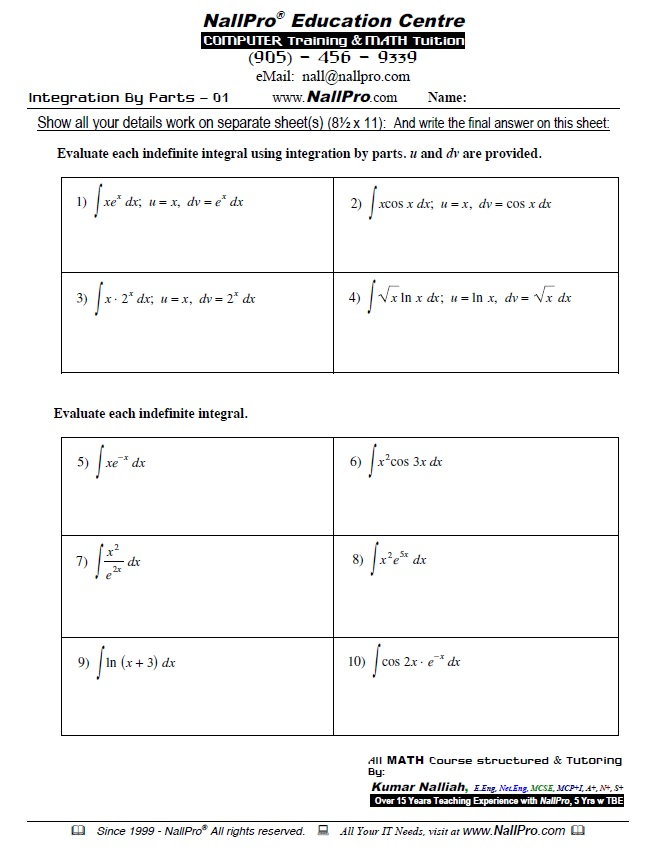Work Sheet 4PDF Printable version

You Will Learn...
 In Advanced Functions...(Course Breakdown) Functions: Characteristics and Properties Functions Exploring Absolute Value Properties of Graphs of Functions Sketching Graphs of Functions Inverse Relations Piecewise Functions Exploring Operations with Functions Functions: Understanding Rates of Change Determining Average Rate of Change Estimating Instantaneous Rates Using Rates of Change Solving Problems Involving Rates of Change Polynomial Functions Exploring Polynomial Functions Characteristics of Polynomial Functions Properties of Graphs of Functions Polynomial Functions in Factored Form Transformations of Cubic and Quartic Functions Dividing Polynomials Factoring Polynomials Factoring a Sum or Difference of Cubes Polynomial Equations and Inequalities Solving Polynomial Equations Solving Linear Inequalities Solving Polynomial Inequalities Rates of Change in Polynomial Functions Rational Functions, Equations, and Inequalities Graphs of Reciprocal Functions Exploring Quotients of Polynomial Functions Graphs of Rational Functions of the Form f(x) Solving Rational Equations Solving Rational Inequalities Rates of Change in Rational Functions Trigonometric Functions Radian Measure Radian Measure and Angles on the Cartesian Plane Exploring Graphs of the Primary Trigonometric Functions Transformations of Trigonometric Functions Exploring Graphs of the Reciprocal Trigonometric Functions Modelling with Trigonometric Functions Rates of Change in Trigonometric Functions Trigonometric Identities and Equations Exploring Equivalent Trigonometric Functions Compound Angle Formulas Double Angle Formulas Proving Trigonometric Identities Solving Linear Trigonometric Equations Solving Quadratic Trigonometric Equations Exponential and Logarithmic Functions Exploring the Logarithmic Function Transformations of Logarithmic Functions Evaluating Logarithms Laws of Logarithms Solving Logarithmic Equations Solving Problems with Exponential and Logarithmic Functions Rates of Change in Exponential and Logarithmic Functions In Data Management...(Course Breakdown) Tools for Data Management Statistics of One/Two Variable Permutations and Organized Counting Combinations and the Binomial Theorem Probability Distributions The Normal Distribution In Calculus...(Course Breakdown) Limits The Limit of a Function Properties of Limits Limit of continuity at a point One-Side Limits Limit as x Approaches to Infinity Differential calculus First Principles Rules of Power, Sum and Difference Product Rule and Quotient Rule Chain Rule Derivative of Trigonometric Functions Derivative of Exponential Functions Derivative of The Natural Log Functions Implicit Differentiation Higher Order Derivative Differential Calculus and Curve Sketching Tangents and Normals Curve Sketching - Increasing and Decreasing Functions Curve Sketching - Global Maxima and Minima The Second Derivative and its Application Rational Functions - Sketching the Graph Applications of Differential Calculus Rates Of Change Applied Rates of Change Kinematics - Motion Along a Straight Line Applied Maxima and Minima Problems Integration and Its Applications Antidifferentiation & The Indefinite Integral Determining The Indefinite Integral The Definite Integral Applications of Integration Introduction to the Area Beneath a Curve The Definite Integral and Areas Applications to Kinematics In Vectors...(Course Breakdown) An Introduction to Vectors Addition and Subtraction of Vectors Multiplication of a Vector by a Scalar Properties of Vectors Vectors in R2 and R3 Operations with Algebraic Vectors in R2 and R3 Linear Combinations and Spanning Sets Applications of Vectors Vectors as Forces Velocity The Dot Product of Two Geometric/Algebraic Vectors Scalar and Vector Projections The Cross Product of Two Vectors Applications of the Dot Product and Cross Product Equations of Lines and Planes Vector and Parametric Equations of a Line in R2 Cartesian Equation of a Line Vector, Parametric, and Symmetric Equations of a Line in R3 Vector and Parametric Equations of a Plane The Cartesian Equation of a Plane Sketching Planes in R3 Extension Concepts...(Course Breakdown) Complex Numbers Integration By Substitution Integration by Parts Integration ApplicationsRegister ON-LINE